The Megaminx Pattern Archive

# The Megaminx Pattern Archive

The megaminx is a rubikized platonic dodecahedron

U Face = Red, L Face = Orange, F Face = Blue, R Face = Yellow

M1 10 spot...(L+ R- C_U-)^36
The 10 spot is Order 5 because it rotates the centres about a face
Here L and R refer to opposite sides of the dodecahedron.

In this case L and R are not separated by a face, as are the sequences below.
Note the centre pieces are rotating in a counter-clockwise direction.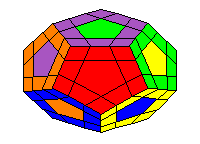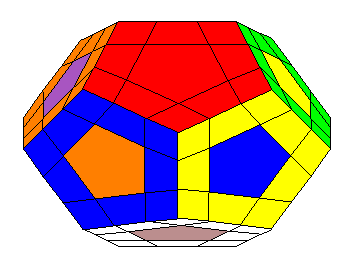M2 3 Cycle...(uf, lf, rf) R+ F+ U+ F- U- R- L- U- F- U+ F+ L+...(12f, 12u)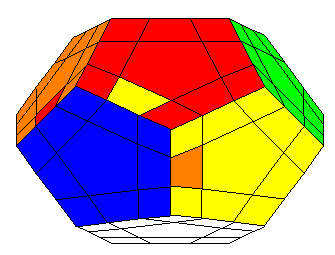M3a 2 flip...(ul, uf) R- F- U+ L- U- L+ F+ R+ L+ F+ U- R+ U+ R- F- L-...(16f, 16u)M4 12 spot type a...(L+ R- C_U-)^36 C_F- (L+ R- C_U-)^36
The 12 spot type a is Order 3 since it rotates the centres about a corner
Like M1, here L and R refer to opposite sides.
The operator C_F- means "rotate the dodecahedron counter-clockwise about face F".
This face ends up with being blue with the red centre.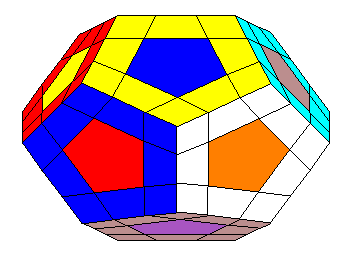m5 12 spot type b...(L+ R- C_U-)^36 C_F- (L+ R- C_U-)^36 C_F+ (L+ R- C_U-)^36
The 12 spot type b is Order 2 since it rotates the centres about an edge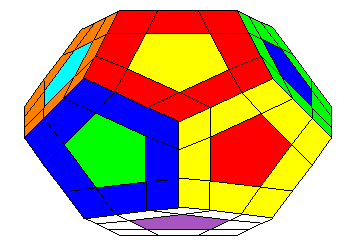In summary, there are twenty-four 10 spots, thirty-five 12 spots and one 0 spot
24 + 35 + 1 = 60 which account for all 60 orientations in space of the megaminx
The thirty-five 12 spots come in 2 types, twenty of order 3 and fifteen of order 2

Credits: The pictures above were created using the Maple package mega.mpl created by Professor David Joyner and the operators of Mark Longridge

Back to Main